Courses

# Principle Of Effective Stress, Capillarity & Permeability - 1

## 10 Questions MCQ Test Mock Test Series for Civil Engineering (CE) GATE 2020 | Principle Of Effective Stress, Capillarity & Permeability - 1

Description
This mock test of Principle Of Effective Stress, Capillarity & Permeability - 1 for Civil Engineering (CE) helps you for every Civil Engineering (CE) entrance exam. This contains 10 Multiple Choice Questions for Civil Engineering (CE) Principle Of Effective Stress, Capillarity & Permeability - 1 (mcq) to study with solutions a complete question bank. The solved questions answers in this Principle Of Effective Stress, Capillarity & Permeability - 1 quiz give you a good mix of easy questions and tough questions. Civil Engineering (CE) students definitely take this Principle Of Effective Stress, Capillarity & Permeability - 1 exercise for a better result in the exam. You can find other Principle Of Effective Stress, Capillarity & Permeability - 1 extra questions, long questions & short questions for Civil Engineering (CE) on EduRev as well by searching above.
QUESTION: 1

### In a falling head permeability test on a soil, the time taken for the head to fall from h0 to h1 is t. The test is repeated with same initial head h0, the final head h' is noted in time t/2. Which one of the following equations gives the relation between h', h0 and h1?

Solution: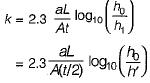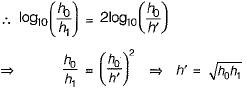QUESTION: 2

### Approximate ratios of the permeabilities of two clean soils having D10 = 0,6 mm and D10 = 0,3 mm respectively is

Solution:

Ratio of permeabilities of the two soils is given by,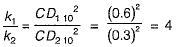QUESTION: 3

### Which one of the following expressions represents the shear strength of soil at point X? Angle of shear resistance of soil is φ and symbols Yw' Ysat' Ysub' Ydry stand for unit weights of water, saturated soil, submerged soil and dry soil respectively.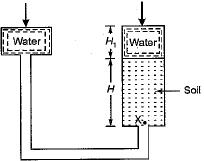Solution:

As the free water surface on both sides is same so there is no flow occurring through soil. Thus effective stress at X,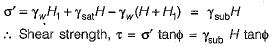QUESTION: 4

A stratified soil deposit has three layers of thicknesses: z1 = 4, z2 - 1, z3 = 2 units and the corresponding permeabilities of k1 = 2, k2 = 1 and k3 = 4 units, respectively. The average permeability perpendicular to the bedding planes will be

Solution: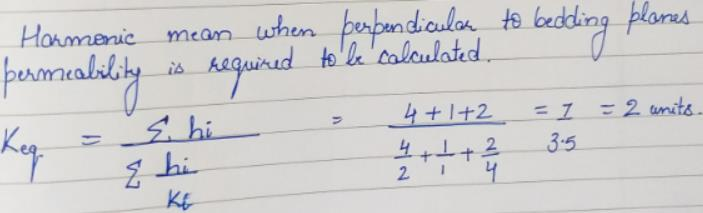QUESTION: 5

In the case of stratified soil layers, the best equation that can be adopted for computing the pressure distribution is

Solution:

Westergaard’s equation is applicable for non-isotropic condition commonly encountered in sedimentary stratified deposits.

QUESTION: 6

The permeability of a soli sample varies (Where d = grain size)

Solution: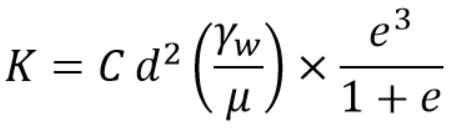K=Cⅆ2 (γ_w/μ)×ⅇ3/(1+ⅇ)
k- permeability; d – dia. of soil particles; e- void ratio;
μ – Viscocity of fluid

QUESTION: 7

In the situation shown in the given figure, the coefficient of permeability of the soil is 10-3cm/s. The discharge in one minute will be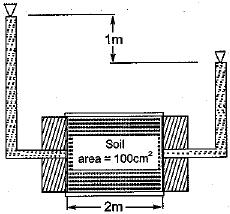Solution:

Discharge = Permeability x Area x Head/ Length= 10-3 x 100 x (1/2) = 1/20 cm3/s
Therefore, volume flown in 1 minute = 60 seconds
60/20 cm3  = 3 cm3 = 3ml

QUESTION: 8

Effective stress in a soil is the stress carried by the

Solution:

Effective stress is a function of the particle contact force.
Pore water pressure acts uniformly on all sides of the particle and does not cause the soil particles to press against each other.

QUESTION: 9

The coefficient of permeability increases when

Solution: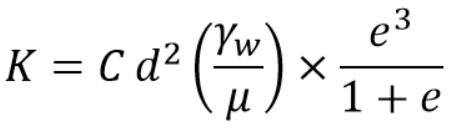K=Cⅆ2 (γ_w/μ)×ⅇ3/(1+ⅇ)
k- permeability; d – dia. of soil particles; e- void ratio;
μ – Viscocity of fluid
Viscocity decreases permeability increases

QUESTION: 10

Which of the following methods is best suited for the determination of permeability of coarse grained soils?

Solution:

Coarse Grained Soil- Constant Head Method
Fine Grained Soil- Falling Head Method